What is Stereochemistry?

What is Stereochemistry?

Key Terms
• Chirality
• Chiral
• Achiral
• Enantiomer
• Chirality center
• Fischer projection
• Cahn-Ingold-Prelog system
• Optical activity

Objectives

• Determine whether a molecule is chiral
• Create Fischer projections for molecules
• Use the Cahn-Ingold-Prelog priority system to determine the sense of chirality
• Recognize that chirality causes optical activity

As molecules get more and more complex, their three-dimensional structure often becomes more complex as well-for instance, the folding of proteins is often extremely convoluted. But even simple molecules can vary in their spatial (three-dimensional) arrangement; these arrangements may affect how a molecule behaves, so appreciating some basic principles of stereochemistry is crucial to a full grasp of organic chemistry.

Thus far, we have seen some examples of how molecules can differ in three dimensions; for instance, the placement of substituent groups around the double bond of an alkene (cis versus trans) is not arbitrary, and different placements yield different molecules with different characteristics. We will focus on one particular aspect of the three-dimensional structure of molecules: chirality.

Chirality

Consider a one-carbon molecule that is substituted with three halogens and one hydrogen atom.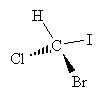Does the placement of the substituent atoms make any difference? To answer this question, let's swap two of the atoms (chlorine and bromine) and then compare the original molecule with the new (swapped) molecule.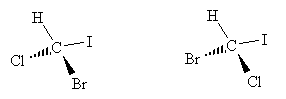The chemical formulas of both molecules are the same: CHBrClI. We cannot, however, take the new molecule and superimpose it exactly over the old molecule. By rotating each molecule slightly, we can show that these two molecules are in fact mirror images of one another.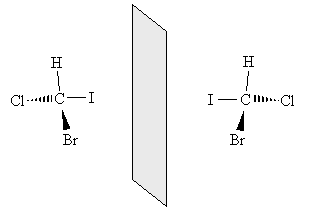Thus, these two molecules have a fundamentally different arrangement of their constituent atoms: they cannot be superimposed through some combination of rotations and translations. This characteristic is called chirality, and a molecule that exhibits chirality is said to be chiral. Molecules that are not chiral are called achiral (for instance, methane is achiral). Two molecules that are mirror images of one another (for instance, the two example molecules shown above) are called enantiomers.

Chiral molecules can generally be identified as compounds in which a carbon atom is bound to four different groups (as in the molecule above). In such a case, mirror images of the molecule cannot be superimposed on each other. The "central" carbon in these molecules is called a chirality center.

Practice Problem: Determine if the molecule 2-methyl-2-chloropentane is chiral, and if so, find its chirality center.

Solution: First, draw the molecule 2-methyl-2-chloropentane.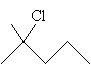Note that each carbon in the pentane chain-except the second carbon-has at least two hydrogen atoms, as does the methyl group attached to the carbon at position 2. The carbon at position 2 is attached to a chlorine atom, a propyl group, and two methyl groups. Thus, no carbon atom in the molecule can qualify as a chirality center (four different substituent groups), so the molecule is achiral.

Practice Problem:
Determine if the molecule 1-chloro-1-fluoropropane is chiral, and if so, find its chirality center.

Solution: Again, start by drawing the molecule.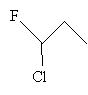The carbon atom at position 1 is attached to a fluorine, a chlorine, a hydrogen, and an ethyl group. Thus, because these groups are all different, 1-chloro-1-fluoropropane is a chiral molecule with the carbon at position 1 being the chirality center.

Fischer Projections

Drawing chiral molecules can be a little time consuming, and sometimes confusing, when using lines and wedges to represent bonds in three dimensions, as with the example molecule we used above.A simpler approach would be to just use lines following a convention that accurately maintains the sense of chirality. One approach is the Fischer projection, which represents chiral molecules using a simple cross structure.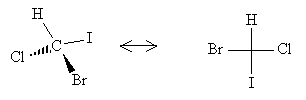To form a Fischer projection, orient the molecule (in your head, if not on paper) so that the vertical bonds are directed below the plane of the page and the horizontal bonds are directed above the plane of the page. Then, replace the wedges with the cross, leaving out the carbon at the chirality center.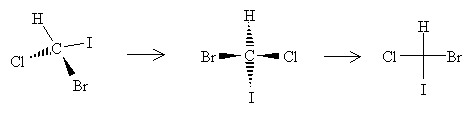For molecules that include a carbon chain, the chain should be oriented vertically with the lowest-position-number carbon at the top. Thus, for instance, 2-chlorobutane should have either of the two Fischer projections below, depending on the chirality of the molecule.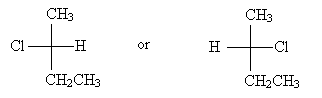Practice Problem: Draw a Fischer projection for 2-iodohexane.

Solution:First, draw 3-iodohexane.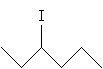The chirality center is the carbon at position 3. We can more easily identify substituent groups and then draw the Fischer projection by writing out these groups instead of using the skeleton drawing. Either one of the two molecules shown below (mirror images) is acceptable, since we have not specified one or the other.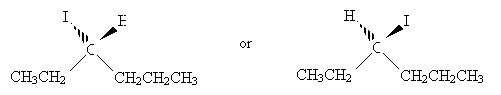Orient the molecules so that the vertical bonds go into the page and the horizontal bonds come out of the page. Make sure that the carbon at position 1 is at the top of the drawing. Next, draw the Fischer projection.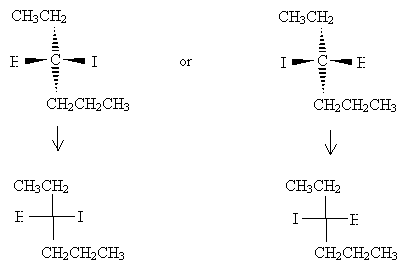Note that the lowest-number carbon appears at the top of the Fischer projection, and the remainder of the hexane chain is at the bottom.

Identifying Chirality

To differentiate two Fischer projections (or, more broadly, the two mirror images of any chiral molecule), we need a systematic way to classify them. A chiral molecule has only one mirror image, so we can classify chiral molecules as being one of two types (what we might call left handed and right handed). First, we must have a system of classification for constituent groups, then we must classify their arrangement in the molecule.

We can classify constituent groups according to the Cahn-Ingold-Prelog system, which ranks a group by atomic number. The rules for this system are summarized below.

1.      Atoms with higher atomic number have priority over those with a lower atomic number (e.g., iodine has priority over chlorine).

2.      Work outward from the chirality center, comparing the atoms directly attached to the chirality center first.

3.      After comparing one set of atoms, next compare all of the atoms directly attached to that set.

4.      Repeat steps 2 and 3 as necessary.

Remark 1. Atoms attached by double bonds count twice.

Consider the example below.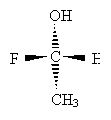To apply the Cahn-Ingold-Prelog rules, start with each atom bound to the chirality center: O, H, F, and C. By atomic number, the order in decreasing priority is F, O, C, H. But what about a slightly more complicated situation?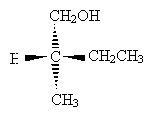Carbon takes priority over hydrogen, but we must proceed further. The carbon in the methyl group only has hydrogen substituents, whereas the carbon in the methyl group is attached to another carbon. Thus, the ethyl group takes priority over the methyl group. But the carbon in the CH2OH group is bound to an oxygen atom, which even beats the ethyl group. Thus, the order of decreasing priority is CH2OH, CH2CH3, CH3, H.

But how does this help determine chirality? We can use these priority rules to systematically identify chirality according to the following rules for a chiral molecule, where the chirality center is identified.

1.      Find the lowest-priority substituent of the chirality center; draw the molecule so that this substituent points into the plane of the page.

2.      Determine the direction (clockwise or counterclockwise) of decreasing priority (highest to second highest to lowest) for the remaining groups. Assign chirality R (from the Latin word for "right") for clockwise and S (from the Latin word for "left") for counterclockwise.

You can remember these assignments using a hand rule: point your thumb in the direction of the lowest-priority group (into the plane of the page) and curl your fingers around your hand. Depending on which hand you choose, your fingers should curl in the direction of decreasing priority for the remaining groups: this is the "handedness" of the molecule. For example, if you use your right hand, your fingers will curl in the clockwise direction, corresponding to chirality R ("right").

Practice Problem:
Determine the sense of chirality (R or S) for the molecule below.Solution: Clearly, hydrogen is the lowest-priority group. If we orient the molecule to direct this group into the plane of the page, we will see the remaining three groups arranged as shown below (the carbon at the chirality center is omitted for simplicity).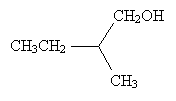The remaining substituent groups decrease in priority as CH2OH, CH2CH3, CH3; this corresponds to the counterclockwise direction, or chirality S. (Try pointing the thumb of your left hand toward the page and notice that your fingers naturally curl in the counterclockwise direction.)

Optical Activity

One of the characteristics of a compound that chirality affects is optical activity, which is a measure of how much the compound causes plane-polarized light to rotate when passed through that compound. Although the direction that a chiral compound rotates plane-polarized light is not related to our above-discussed (and arbitrary) system of distinguishing the sense of chirality, the two different enantiomers of a chiral compound will rotate light in different directions. When present in equal amounts, the effects from the two enantiomers will "cancel" each other, yielding no net rotation. When one amount exceeds the other, however, plane-polarized light will be rotated by some amount related to the concentration of the prevailing enantiomer (relative to that of the lesser enantiomer).

We will not dwell on the mechanics or numerical aspects of optical activity, and optical activity is not the only manifest characteristic of chiral molecules, but it exemplifies the fact that stereochemistry-and, in particular, chirality-has a macroscopic (visible) effect on the behavior of a compound.

Explore